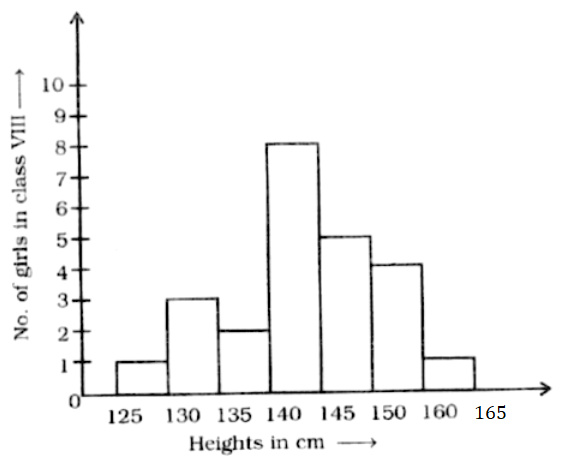Home/Class 8/Maths/

## QuestionMathsClass 8Observe the histogram given above. The number of girls having height $$145\ cm$$ and above is（ ）
A. $$5$$
B. $$10$$
C. $$17$$
D. $$19$$

B
4.64.6## Solution

From the given histogram:
Heights above $$145\ cm$$ are $$150\ cm,160cm$$ and $$165\ cm$$.
So, the number of girls having height $$145\ cm$$ and above is
$$=5+4+1$$
$$=10\$$
Hence, the correct option is B